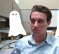## Lyons needs help with a "Frequency Estimation" paper.Started by 6 years ago14 replieslatest reply 6 years ago274 views

Hi Troops.

I'm unable to understand a paper describing what the authors' call a "new frequency estimation algorithm."  For those of you who have plenty of free time at work, and no personal life that would interfere with my request, would you please click the "Download" button at:

https://www.degruyter.com/view/j/wwfaa.2015.1.issu...

and see if you understand the first couple of pages of the frequency estimation paper.  For the life of me I can't figure out the meaning of the authors' Equations (4) & (5).   Thanks!!!

[ - ]By algebraic symbol manipulation, I get equation 4 as

X(e^{jw}) = 1/N * \sum_{n=0}^{L-1} \sum_{k=0}^{N-1} X_N(k) e^{-j(w-2k/N)n}.

The author, however, seems to have applied the \sum_{n=0}^{L-1}

through to the last term e^{-j(w-2k/N)n}, to obtain G(w,k).

I don't yet see how all of those other terms other than n=L cancel to make G(w,k).

I think his aim is padding with zeros for frequency interpolation, many times for many different values of N>=L.  I haven't finished reading the paper, so I don't know if he is merely looking for some N that moves the peak energy close to the center of a frequency bin, or if he develops an equation using the multiple DFT results from many different values of N.

[ - ]Thats right G(w,k) falls out from what you have set up

$$X(e^{jw}) = 1/N * \sum_{n=0}^{L-1} \sum_{k=0}^{N-1} X_N(k) e^{-j(w-2k/N)n}$$

the geometric series of this term

$$\sum_{n=0}^{L-1} e^{-j(w-2k/N)n}$$

is

$$\frac{1 - e^{-j(w-2k/N)L}}{1-e^{-j(w-2k/N)}}$$

or our G(w,k) here.

I'm not sure how multiplying this by the original spectrum allows us to zoom with more resolution. Seems it would just be a cascade of filters at the original resolution...That part seems a little iffy

[ - ]How did you get the fancy graphics?

[ - ]put your latex between double $$[ - ] Reply by February 15, 2018I'm not sure how multiplying this by the original spectrum allows us to zoom with more resolution. Seems it would just be a cascade of filters at the original resolution...That part seems a little iffy N >> L so the resolution is increased. [ - ] Reply by February 15, 2018Lol so this paper is just a very convoluted way of saying 'an N-point DFT much larger than the signal length, L, leads to better spectral resolution' ?? Not very novel research... [ - ] Reply by February 15, 2018There seems to be something more going in in the summation over k to the large value of N >> L. [ - ] Reply by February 15, 2018Not really seeing it. The$$X_{K}(K) G(w,K)

summation would be a point by point multiply. Essentially as they are increasing N they are 'zooming'(but not really) into the bandpass filter region defined by G(w,K). It seems what this affords you is an easy visual evaluation or it would reduce computational complexity of finding the peak for very very large N. It would only need to search the G(w,K) passband instead of iterating over the entire N. But that would only work because you roughly know where the frequency your estimating already is?

[ - ]How G(w,k) falls out of the substitution is still unclear to me. But G(w,k)'s function is basically to create a bandpass filter around a area of w in order to 'zoom' into the band. I'm still unsure how exactly more resolution is obtained while zooming...My intuition says that as it zooms into the band of interest [however that exactly works?] it keeps k (or N) bins in the zoomed in band therefore affording you more spectral resolution.

Some Matlab exploration:

N = 64

L = N

w = 3 % < pi

k = [0:N-1];

Gwk  = (1-exp(-1i*(w - 2*pi*k/N )*L))./(1-exp(-1i*(w-2*pi*k/N)))

plot([0:1/N:1-1/N]*2*pi, 20*log10(abs(Gwk)))

[ - ]For the author's technique to work, N>=L.  Probably N>>L.

[ - ]A little off topic, but here is a way to increase the resolution of your spectrum display:  Steven Kay and Cedric Demeure, “The High-Resolution Spectrum Estimator – a Subjective Entity”, Proceedings IEEE, Vol 72, Dec 1984, pp1815-1816

I use this nonlinear transform to improve resolution of a DFT spectrum to be more like the resolution of a MUSIC spectrum:

Smusic = .01 / (1 - .99*Sdft)

[ - ]Hi John.  You're reminding me that someday I should study the MUSIC frequency estimation process.

[ - ]The math looks kind of bad to me.  As the others have already pointed out, (5) comes from applying the geometric series sum formula to the "n=0 to L-1" summation of the combined exponential terms.  They reverse the order of summation from the substitution without saying so, but that looks alright.

However, from (5) to (6), since they use the "sinc" function instead of the "sin" function, they somehow drop a factor of "L" that should be there.  They also drop a negative sign in the exponent that should be there.

Then from (6) to (7), they somehow think it's okay to factor out a factor of $\pi$ from inside the sinc funtions.  This isn't correct either.

I haven't bothered to study the paper further.

Ced
[ - ]